Скачать презентацию EMT 113 4 ELECTRICAL ENGINEERING TECHNOLOGY Lecturers Ms

• Количество слайдов: 69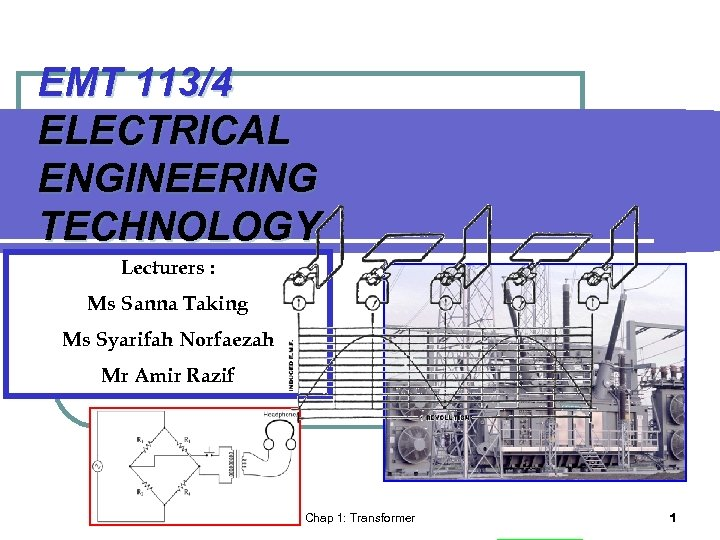EMT 113/4 ELECTRICAL ENGINEERING TECHNOLOGY Lecturers : Ms Sanna Taking Ms Syarifah Norfaezah Mr Amir Razif Chap 1: Transformer 1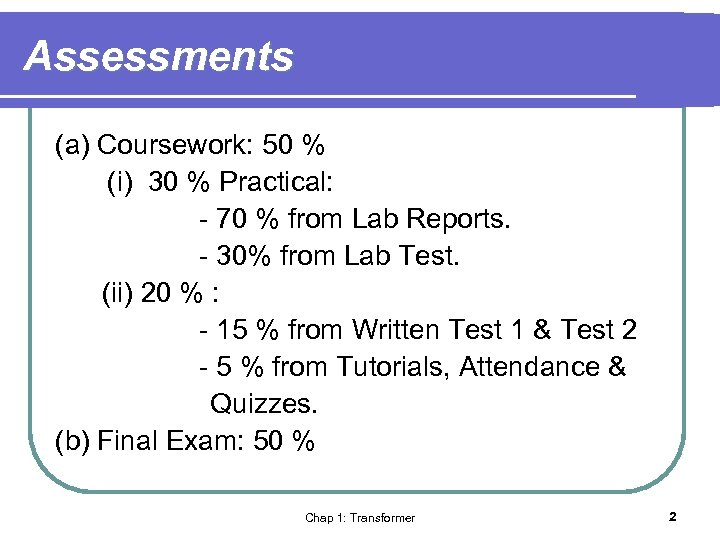Assessments (a) Coursework: 50 % (i) 30 % Practical: - 70 % from Lab Reports. - 30% from Lab Test. (ii) 20 % : - 15 % from Written Test 1 & Test 2 - 5 % from Tutorials, Attendance & Quizzes. (b) Final Exam: 50 % Chap 1: Transformer 2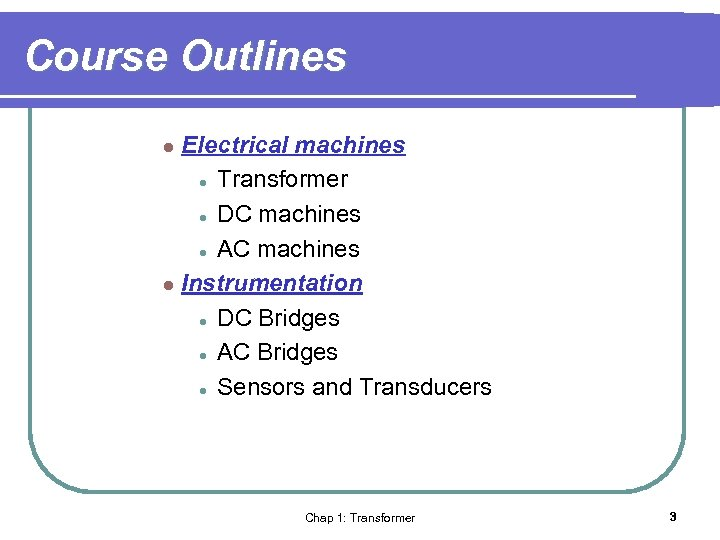Course Outlines Electrical machines l Transformer l DC machines l AC machines l Instrumentation l DC Bridges l AC Bridges l Sensors and Transducers l Chap 1: Transformer 3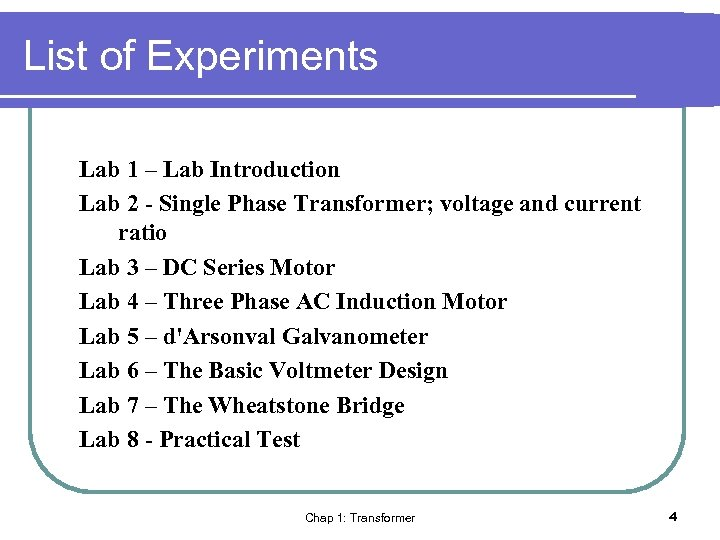List of Experiments Lab 1 – Lab Introduction Lab 2 - Single Phase Transformer; voltage and current ratio Lab 3 – DC Series Motor Lab 4 – Three Phase AC Induction Motor Lab 5 – d'Arsonval Galvanometer Lab 6 – The Basic Voltmeter Design Lab 7 – The Wheatstone Bridge Lab 8 - Practical Test Chap 1: Transformer 4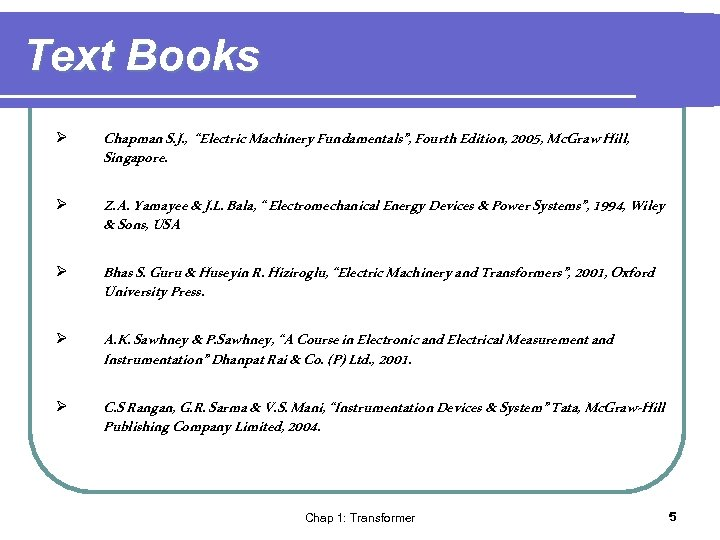Text Books Ø Chapman S. J. , “Electric Machinery Fundamentals”, Fourth Edition, 2005, Mc. Graw Hill, Singapore. Ø Z. A. Yamayee & J. L. Bala, “ Electromechanical Energy Devices & Power Systems”, 1994, Wiley & Sons, USA Ø Bhas S. Guru & Huseyin R. Hiziroglu, “Electric Machinery and Transformers”, 2001, Oxford University Press. Ø A. K. Sawhney & P. Sawhney, “A Course in Electronic and Electrical Measurement and Instrumentation” Dhanpat Rai & Co. (P) Ltd. , 2001. Ø C. S Rangan, G. R. Sarma & V. S. Mani, “Instrumentation Devices & System” Tata, Mc. Graw-Hill Publishing Company Limited, 2004. Chap 1: Transformer 5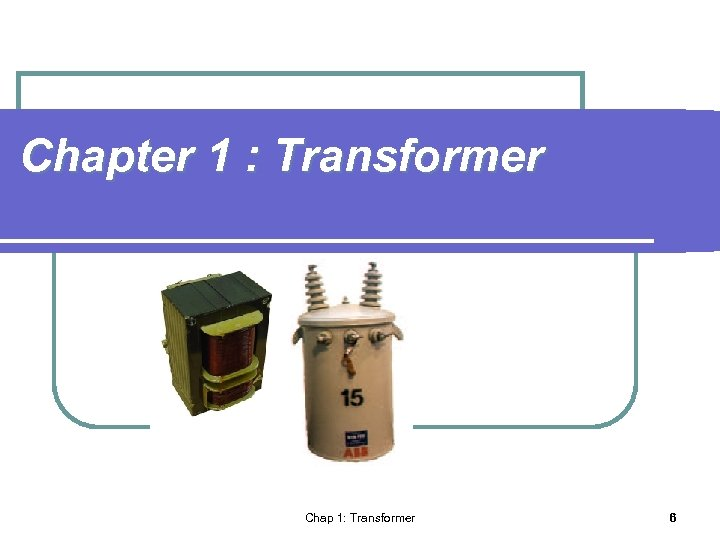Chapter 1 : Transformer Chap 1: Transformer 6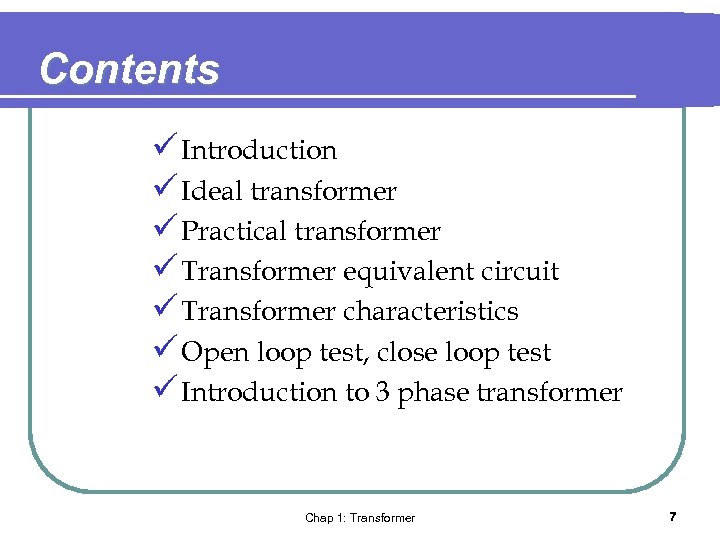Contents ü Introduction ü Ideal transformer ü Practical transformer ü Transformer equivalent circuit ü Transformer characteristics ü Open loop test, close loop test ü Introduction to 3 phase transformer Chap 1: Transformer 7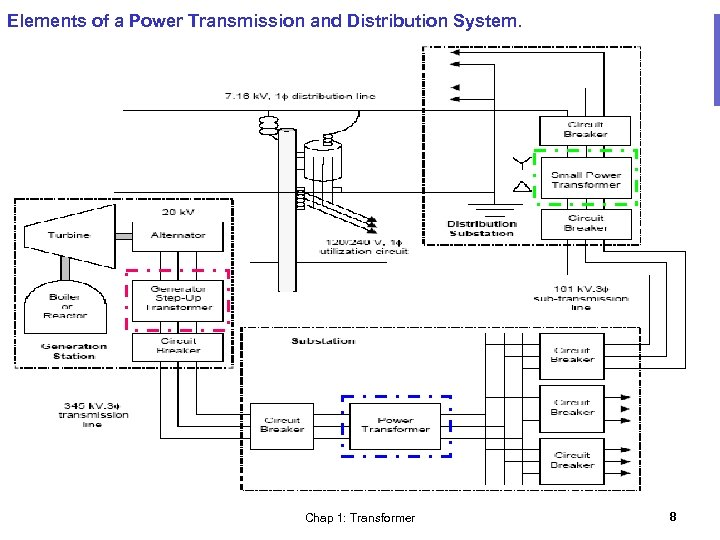Elements of a Power Transmission and Distribution System. Chap 1: Transformer 8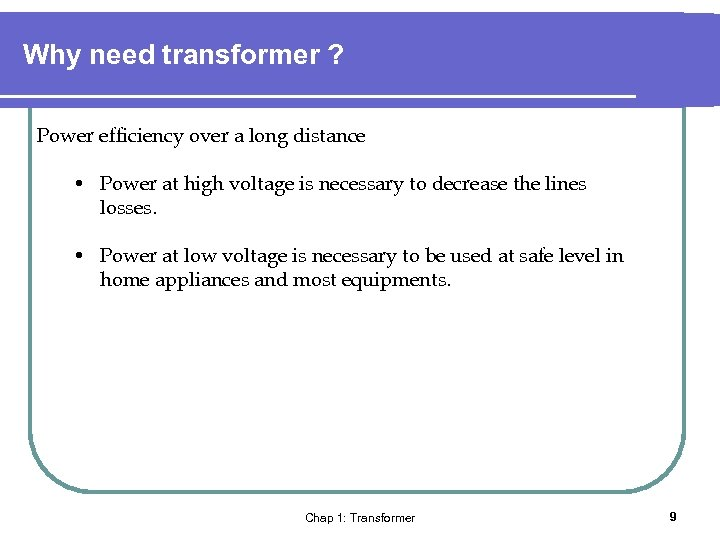Why need transformer ? Power efficiency over a long distance • Power at high voltage is necessary to decrease the lines losses. • Power at low voltage is necessary to be used at safe level in home appliances and most equipments. Chap 1: Transformer 9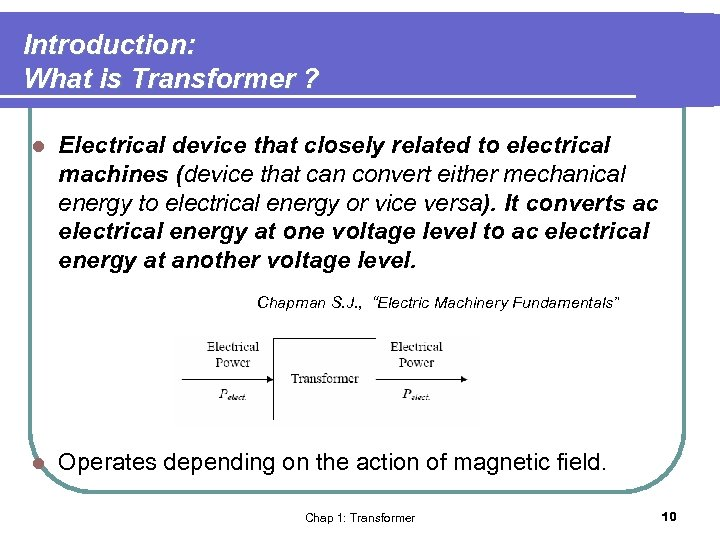Introduction: What is Transformer ? l Electrical device that closely related to electrical machines (device that can convert either mechanical energy to electrical energy or vice versa). It converts ac electrical energy at one voltage level to ac electrical energy at another voltage level. Chapman S. J. , “Electric Machinery Fundamentals” l Operates depending on the action of magnetic field. Chap 1: Transformer 10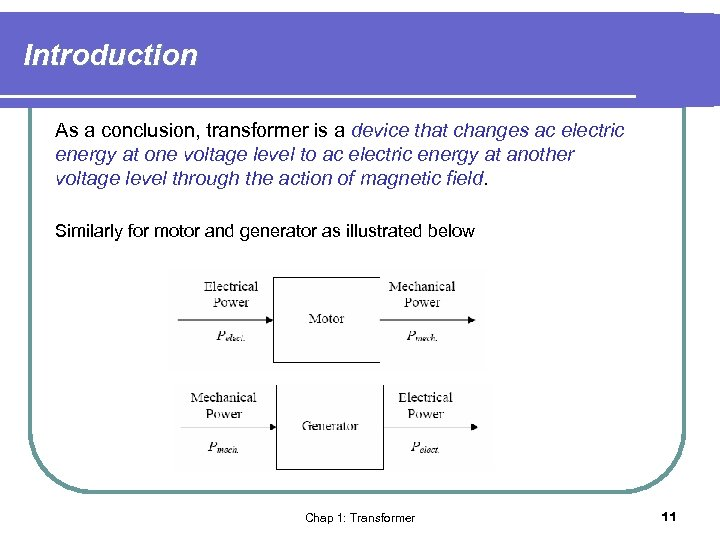Introduction As a conclusion, transformer is a device that changes ac electric energy at one voltage level to ac electric energy at another voltage level through the action of magnetic field. Similarly for motor and generator as illustrated below Chap 1: Transformer 11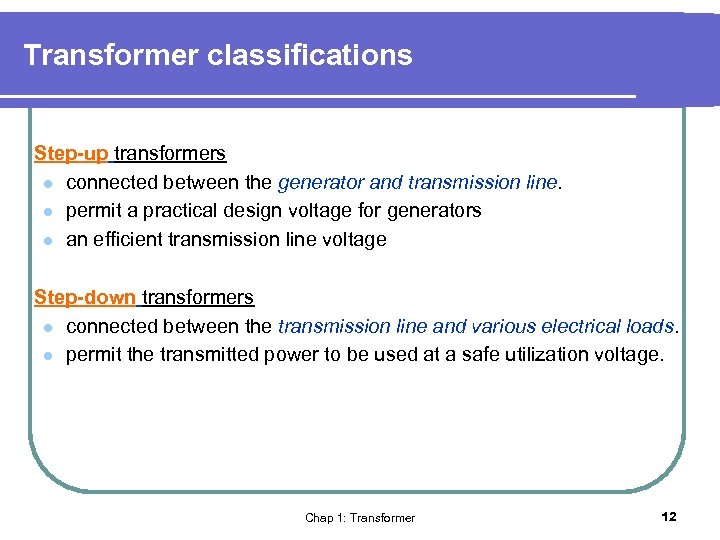Transformer classifications Step-up transformers l connected between the generator and transmission line. l permit a practical design voltage for generators l an efficient transmission line voltage Step-down transformers l connected between the transmission line and various electrical loads. l permit the transmitted power to be used at a safe utilization voltage. Chap 1: Transformer 12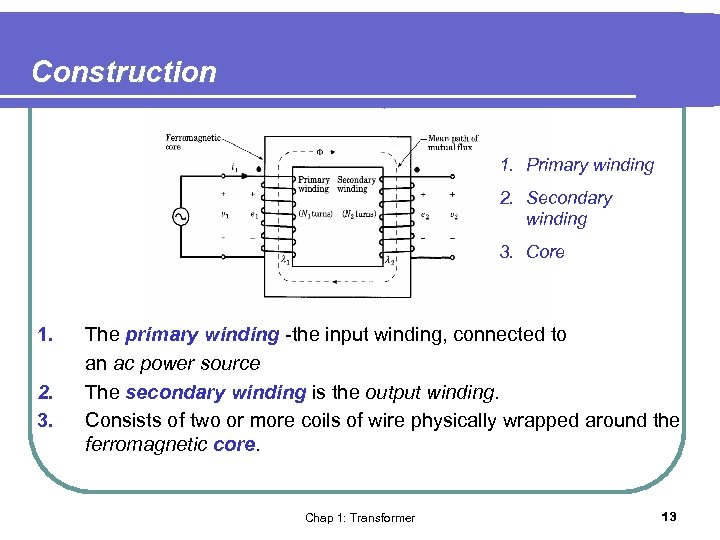Construction 1. Primary winding 2. Secondary winding 3. Core 1. 2. 3. The primary winding -the input winding, connected to an ac power source The secondary winding is the output winding. Consists of two or more coils of wire physically wrapped around the ferromagnetic core. Chap 1: Transformer 13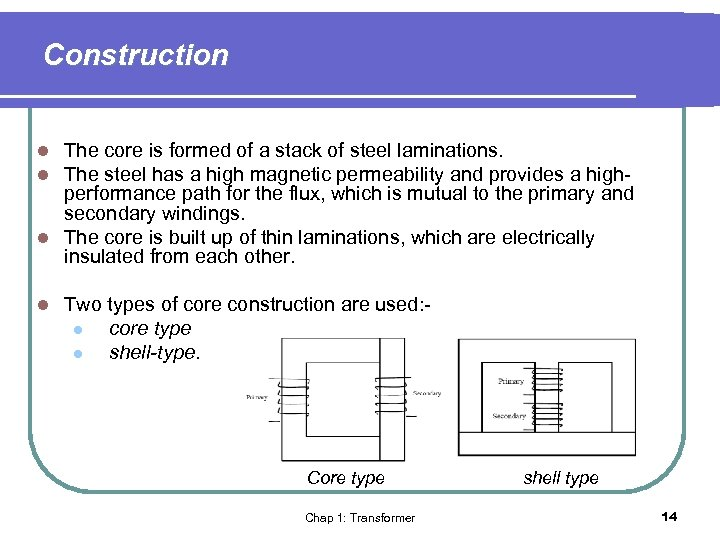Construction The core is formed of a stack of steel laminations. The steel has a high magnetic permeability and provides a highperformance path for the flux, which is mutual to the primary and secondary windings. l The core is built up of thin laminations, which are electrically insulated from each other. l l l Two types of core construction are used: l core type l shell-type. Core type Chap 1: Transformer shell type 14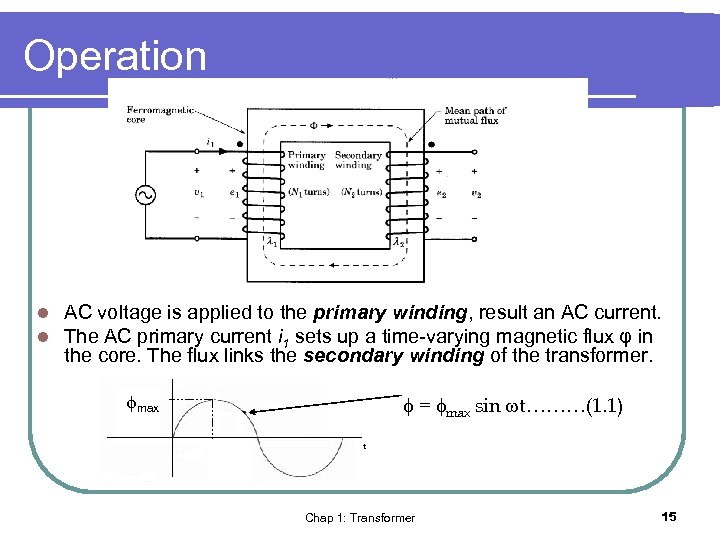Operation l l AC voltage is applied to the primary winding, result an AC current. The AC primary current i 1 sets up a time-varying magnetic flux φ in the core. The flux links the secondary winding of the transformer. max = max sin t………(1. 1) Chap 1: Transformer 15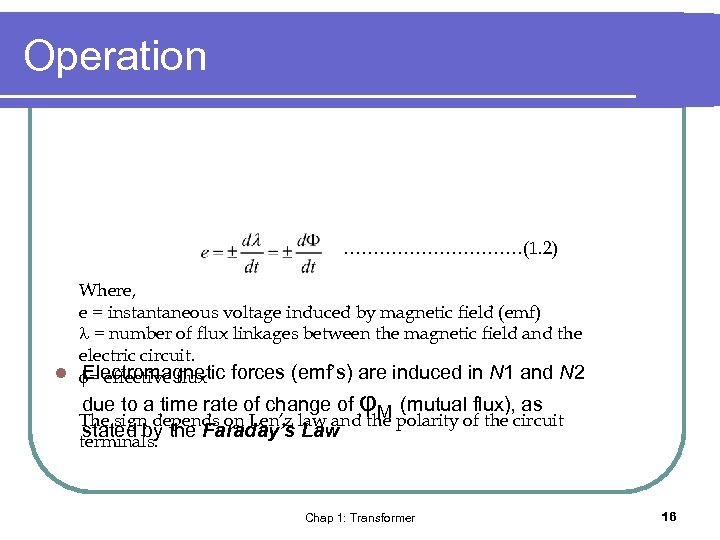Operation ……………(1. 2) Where, e = instantaneous voltage induced by magnetic field (emf) = number of flux linkages between the magnetic field and the electric circuit. l = effective flux forces (emf’s) are induced in N 1 and N 2 Electromagnetic due to a time rate of change of φM (mutual flux), as The sign depends on Len’z law and the polarity of the circuit stated by the Faraday’s Law terminals. Chap 1: Transformer 16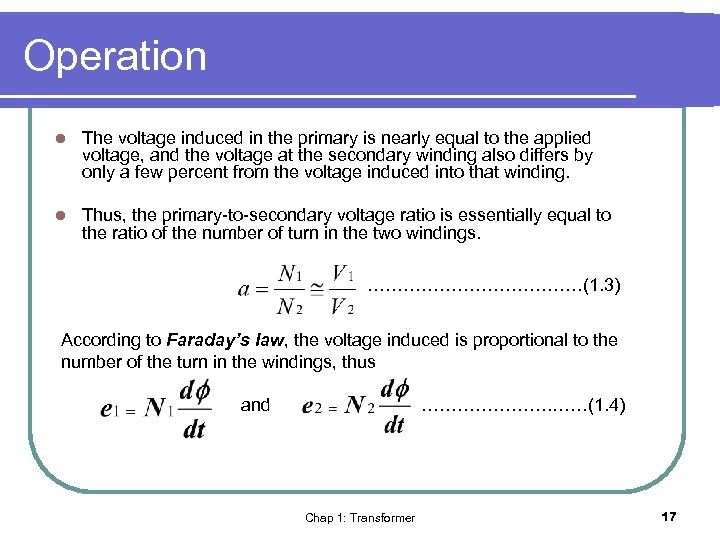Operation l The voltage induced in the primary is nearly equal to the applied voltage, and the voltage at the secondary winding also differs by only a few percent from the voltage induced into that winding. l Thus, the primary-to-secondary voltage ratio is essentially equal to the ratio of the number of turn in the two windings. ………………(1. 3) According to Faraday’s law, the voltage induced is proportional to the number of the turn in the windings, thus and …………………. ……(1. 4) Chap 1: Transformer 17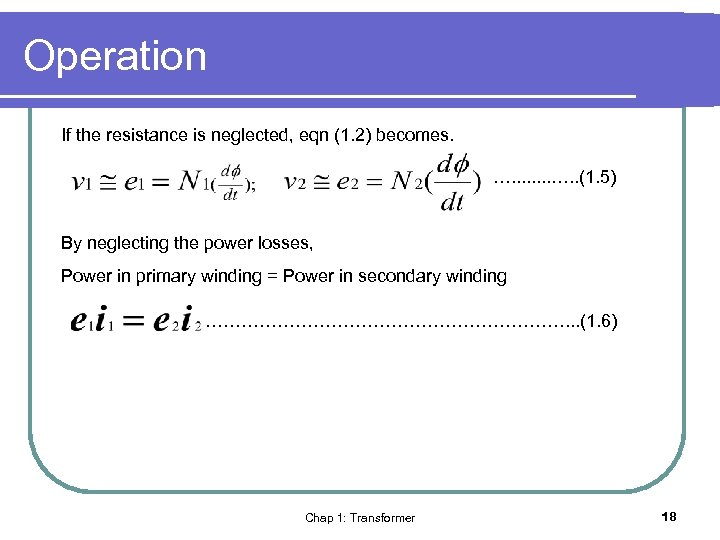Operation If the resistance is neglected, eqn (1. 2) becomes. …. . (1. 5) By neglecting the power losses, Power in primary winding = Power in secondary winding …………………………. . . (1. 6) Chap 1: Transformer 18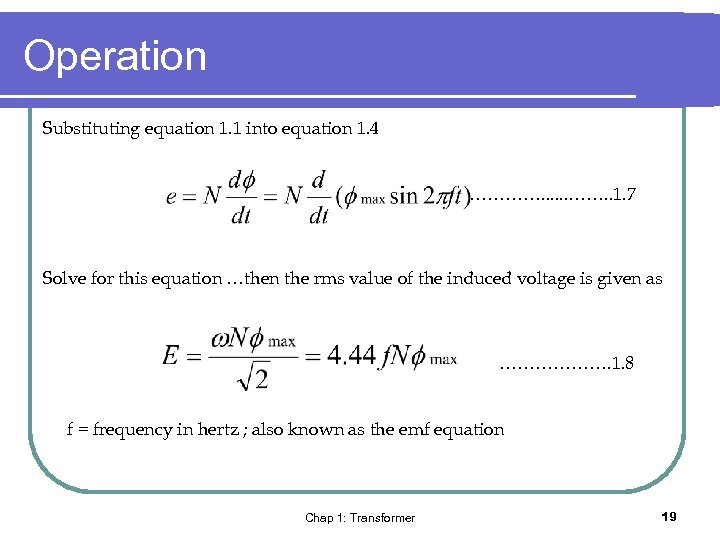Operation Substituting equation 1. 1 into equation 1. 4 …………. . 1. 7 Solve for this equation …then the rms value of the induced voltage is given as ………………. 1. 8 f = frequency in hertz ; also known as the emf equation Chap 1: Transformer 19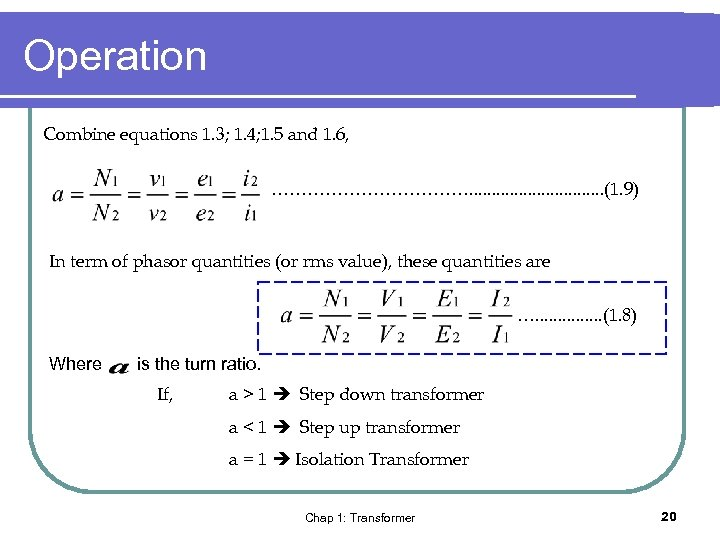Operation Combine equations 1. 3; 1. 4; 1. 5 and 1. 6, ………………. . . . (1. 9) In term of phasor quantities (or rms value), these quantities are …. . . . (1. 8) Where is the turn ratio. If, a > 1 Step down transformer a < 1 Step up transformer a = 1 Isolation Transformer Chap 1: Transformer 20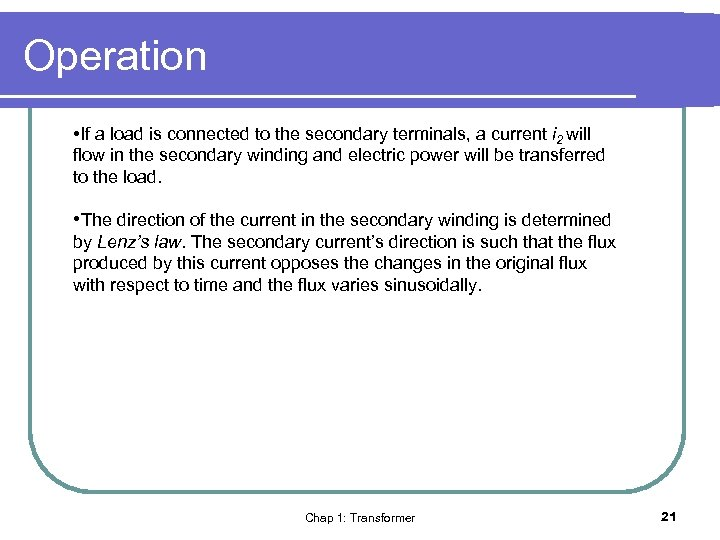Operation • If a load is connected to the secondary terminals, a current i 2 will flow in the secondary winding and electric power will be transferred to the load. • The direction of the current in the secondary winding is determined by Lenz’s law. The secondary current’s direction is such that the flux produced by this current opposes the changes in the original flux with respect to time and the flux varies sinusoidally. Chap 1: Transformer 21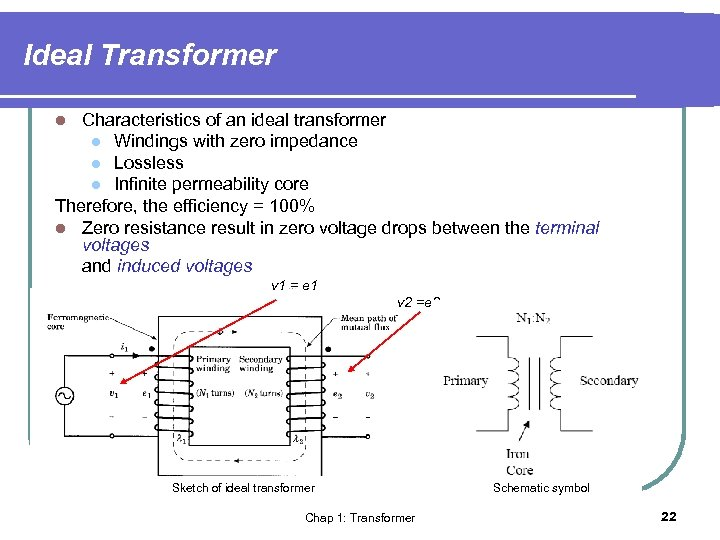Ideal Transformer Characteristics of an ideal transformer l Windings with zero impedance l Lossless l Infinite permeability core Therefore, the efficiency = 100% l Zero resistance result in zero voltage drops between the terminal voltages and induced voltages l v 1 = e 1 v 2 =e 2 Sketch of ideal transformer Chap 1: Transformer Schematic symbol 22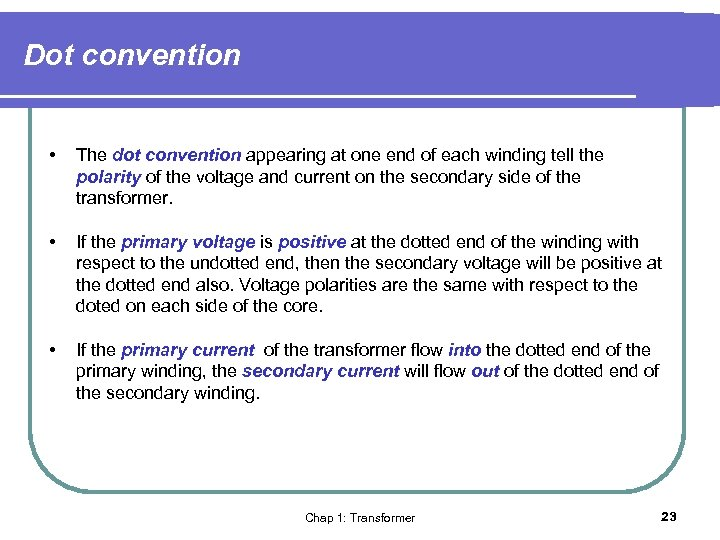Dot convention • The dot convention appearing at one end of each winding tell the polarity of the voltage and current on the secondary side of the transformer. • If the primary voltage is positive at the dotted end of the winding with respect to the undotted end, then the secondary voltage will be positive at the dotted end also. Voltage polarities are the same with respect to the doted on each side of the core. • If the primary current of the transformer flow into the dotted end of the primary winding, the secondary current will flow out of the dotted end of the secondary winding. Chap 1: Transformer 23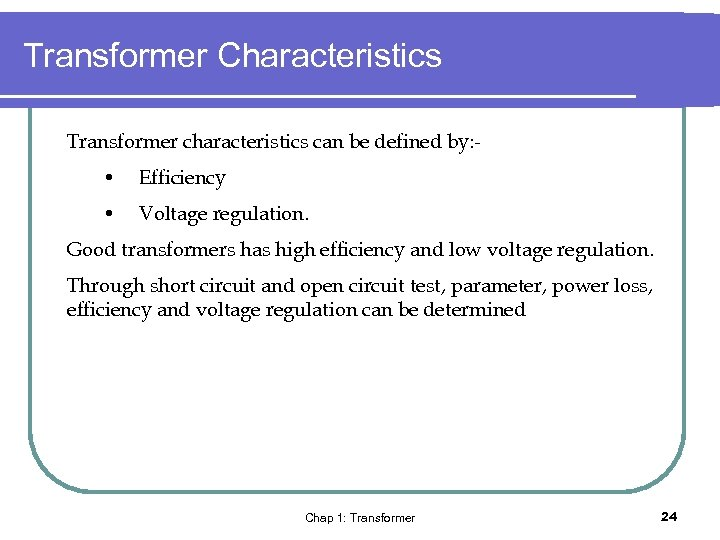Transformer Characteristics Transformer characteristics can be defined by: - • Efficiency • Voltage regulation. Good transformers has high efficiency and low voltage regulation. Through short circuit and open circuit test, parameter, power loss, efficiency and voltage regulation can be determined Chap 1: Transformer 24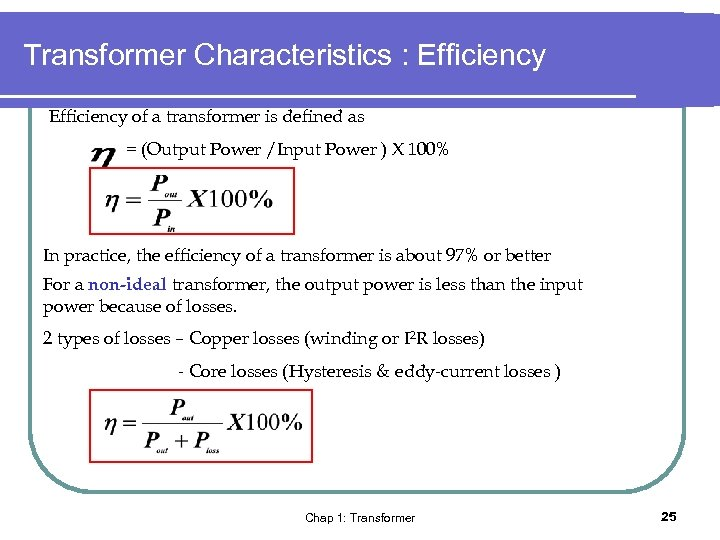Transformer Characteristics : Efficiency of a transformer is defined as = (Output Power /Input Power ) X 100% In practice, the efficiency of a transformer is about 97% or better For a non-ideal transformer, the output power is less than the input power because of losses. 2 types of losses – Copper losses (winding or I 2 R losses) - Core losses (Hysteresis & eddy-current losses ) Chap 1: Transformer 25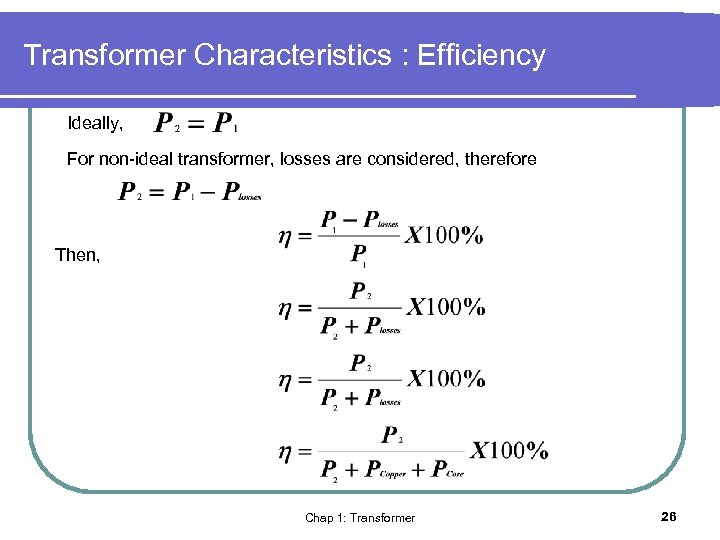Transformer Characteristics : Efficiency Ideally, For non-ideal transformer, losses are considered, therefore Then, Chap 1: Transformer 26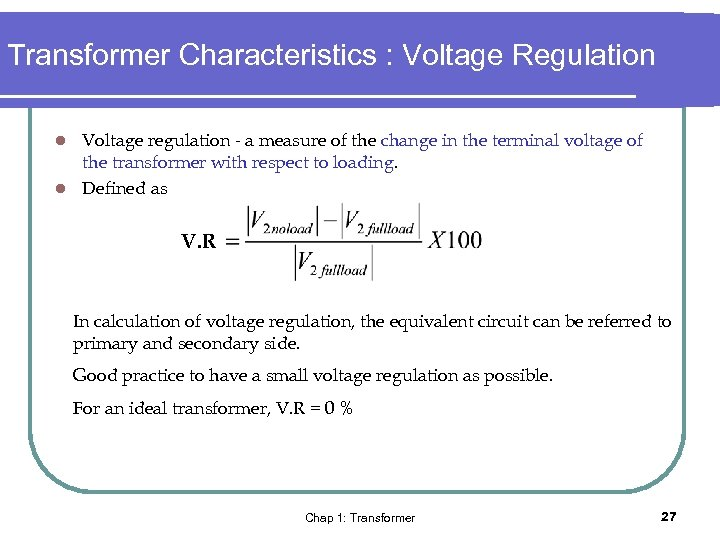Transformer Characteristics : Voltage Regulation Voltage regulation - a measure of the change in the terminal voltage of the transformer with respect to loading. l Defined as l V. R In calculation of voltage regulation, the equivalent circuit can be referred to primary and secondary side. Good practice to have a small voltage regulation as possible. For an ideal transformer, V. R = 0 % Chap 1: Transformer 27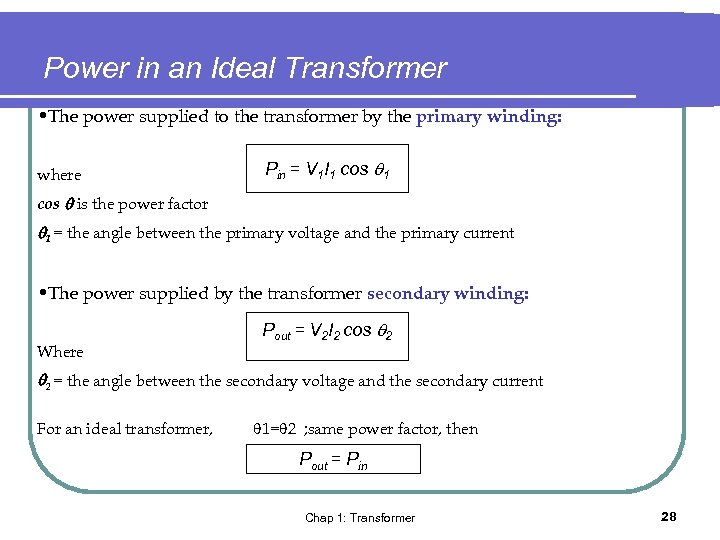Power in an Ideal Transformer • The power supplied to the transformer by the primary winding: where Pin = V 1 I 1 cos is the power factor 1 = the angle between the primary voltage and the primary current • The power supplied by the transformer secondary winding: Where Pout = V 2 I 2 cos 2 2 = the angle between the secondary voltage and the secondary current For an ideal transformer, 1= 2 ; same power factor, then Pout = Pin Chap 1: Transformer 28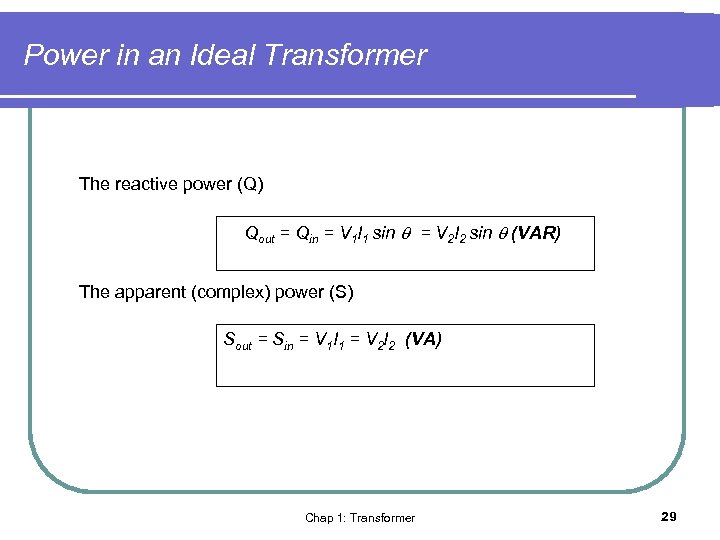Power in an Ideal Transformer The reactive power (Q) Qout = Qin = V 1 I 1 sin = V 2 I 2 sin (VAR) The apparent (complex) power (S) Sout = Sin = V 1 I 1 = V 2 I 2 (VA) Chap 1: Transformer 29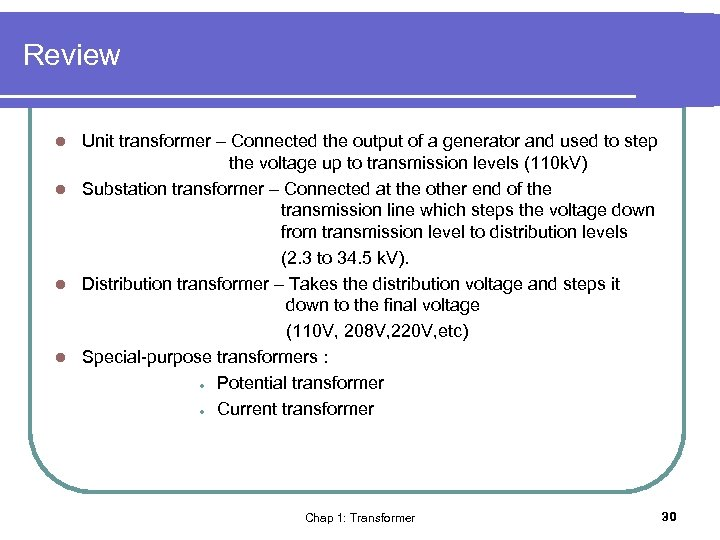Review Unit transformer – Connected the output of a generator and used to step the voltage up to transmission levels (110 k. V) l Substation transformer – Connected at the other end of the transmission line which steps the voltage down from transmission level to distribution levels (2. 3 to 34. 5 k. V). l Distribution transformer – Takes the distribution voltage and steps it down to the final voltage (110 V, 208 V, 220 V, etc) l Special-purpose transformers : l Potential transformer l Current transformer l Chap 1: Transformer 30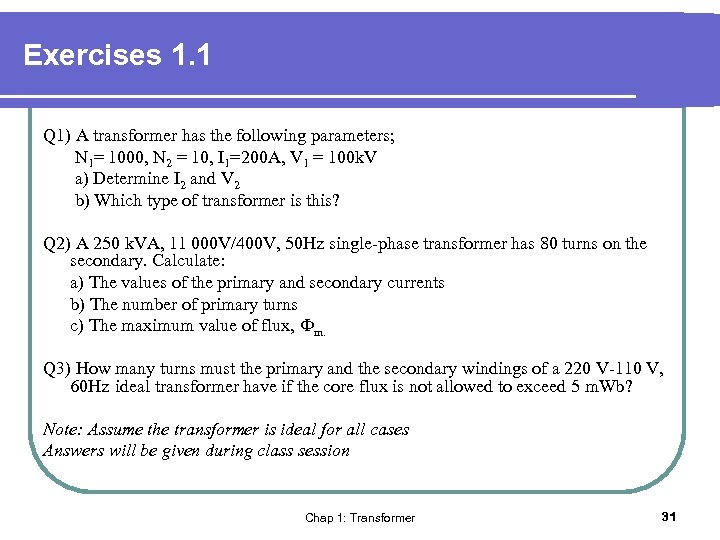Exercises 1. 1 Q 1) A transformer has the following parameters; N 1= 1000, N 2 = 10, I 1=200 A, V 1 = 100 k. V a) Determine I 2 and V 2 b) Which type of transformer is this? Q 2) A 250 k. VA, 11 000 V/400 V, 50 Hz single-phase transformer has 80 turns on the secondary. Calculate: a) The values of the primary and secondary currents b) The number of primary turns c) The maximum value of flux, Фm. Q 3) How many turns must the primary and the secondary windings of a 220 V-110 V, 60 Hz ideal transformer have if the core flux is not allowed to exceed 5 m. Wb? Note: Assume the transformer is ideal for all cases Answers will be given during class session Chap 1: Transformer 31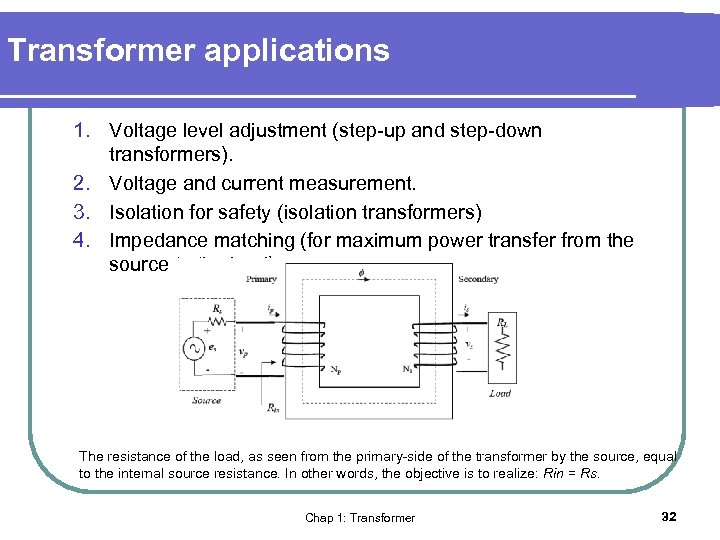Transformer applications 1. Voltage level adjustment (step-up and step-down transformers). 2. Voltage and current measurement. 3. Isolation for safety (isolation transformers) 4. Impedance matching (for maximum power transfer from the source to the load) The resistance of the load, as seen from the primary-side of the transformer by the source, equal to the internal source resistance. In other words, the objective is to realize: Rin = Rs. Chap 1: Transformer 32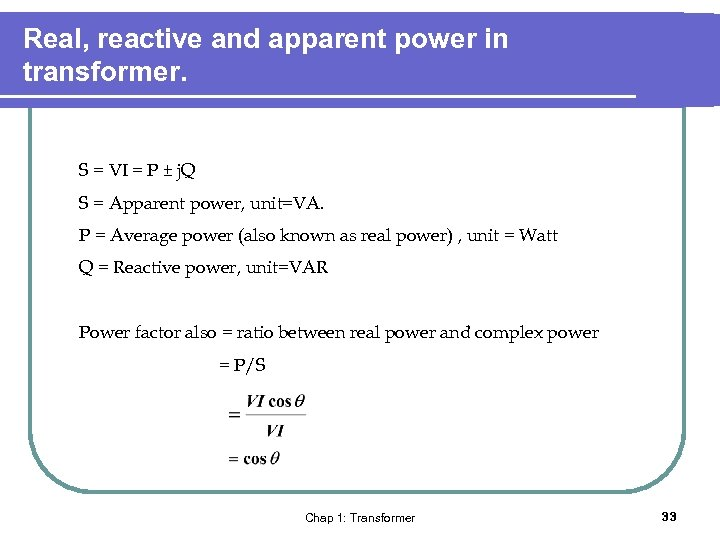Real, reactive and apparent power in transformer. S = VI = P ± j. Q S = Apparent power, unit=VA. P = Average power (also known as real power) , unit = Watt Q = Reactive power, unit=VAR Power factor also = ratio between real power and complex power = P/S Chap 1: Transformer 33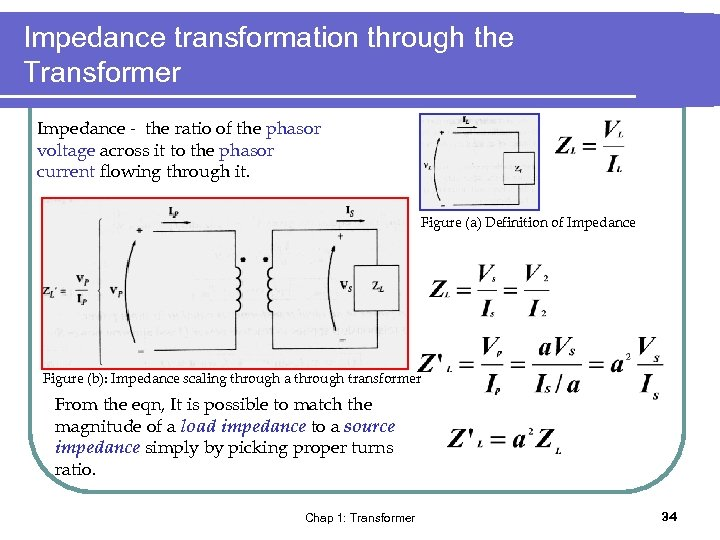Impedance transformation through the Transformer Impedance - the ratio of the phasor voltage across it to the phasor current flowing through it. Figure (a) Definition of Impedance Figure (b): Impedance scaling through a through transformer From the eqn, It is possible to match the magnitude of a load impedance to a source impedance simply by picking proper turns ratio. Chap 1: Transformer 34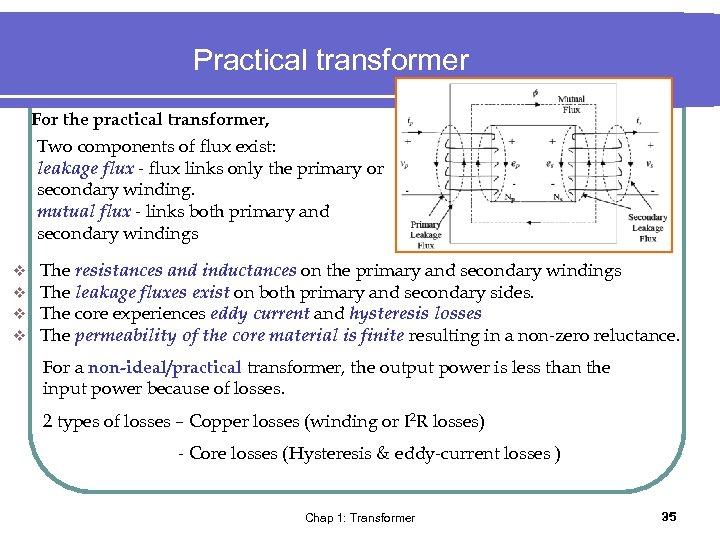Practical transformer For the practical transformer, Two components of flux exist: leakage flux - flux links only the primary or secondary winding. mutual flux - links both primary and secondary windings v v The resistances and inductances on the primary and secondary windings The leakage fluxes exist on both primary and secondary sides. The core experiences eddy current and hysteresis losses The permeability of the core material is finite resulting in a non-zero reluctance. For a non-ideal/practical transformer, the output power is less than the input power because of losses. 2 types of losses – Copper losses (winding or I 2 R losses) - Core losses (Hysteresis & eddy-current losses ) Chap 1: Transformer 35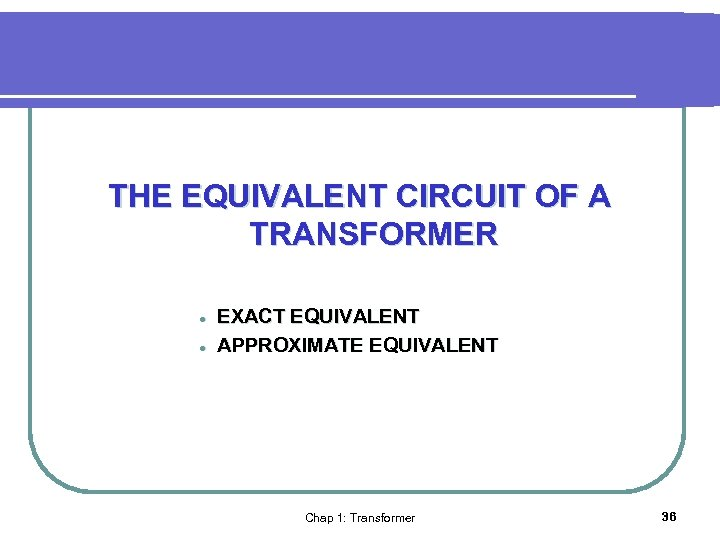THE EQUIVALENT CIRCUIT OF A TRANSFORMER l l EXACT EQUIVALENT APPROXIMATE EQUIVALENT Chap 1: Transformer 36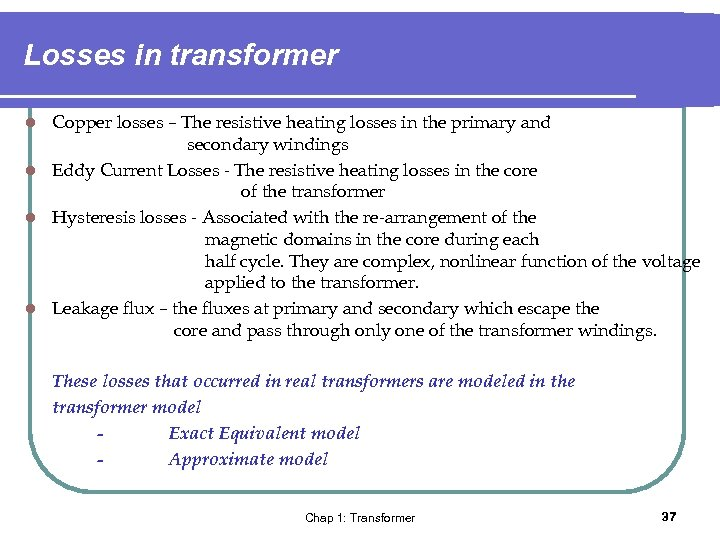Losses in transformer Copper losses – The resistive heating losses in the primary and secondary windings l Eddy Current Losses - The resistive heating losses in the core of the transformer l Hysteresis losses - Associated with the re-arrangement of the magnetic domains in the core during each half cycle. They are complex, nonlinear function of the voltage applied to the transformer. l Leakage flux – the fluxes at primary and secondary which escape the core and pass through only one of the transformer windings. l These losses that occurred in real transformers are modeled in the transformer model Exact Equivalent model Approximate model Chap 1: Transformer 37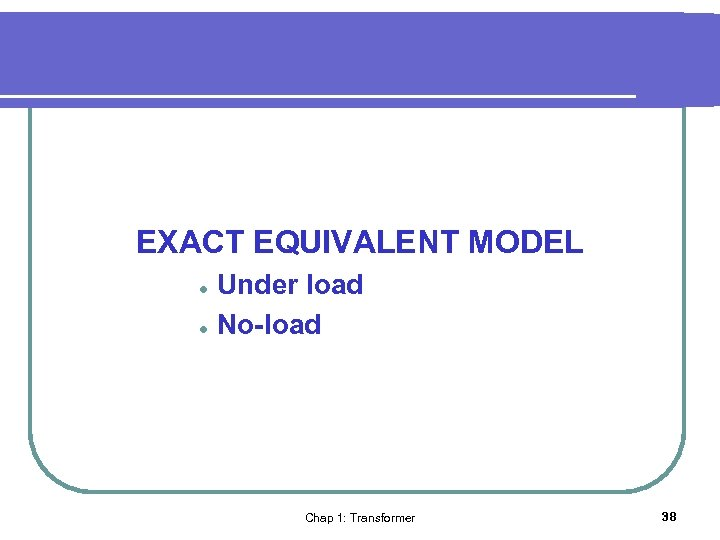EXACT EQUIVALENT MODEL l l Under load No-load Chap 1: Transformer 38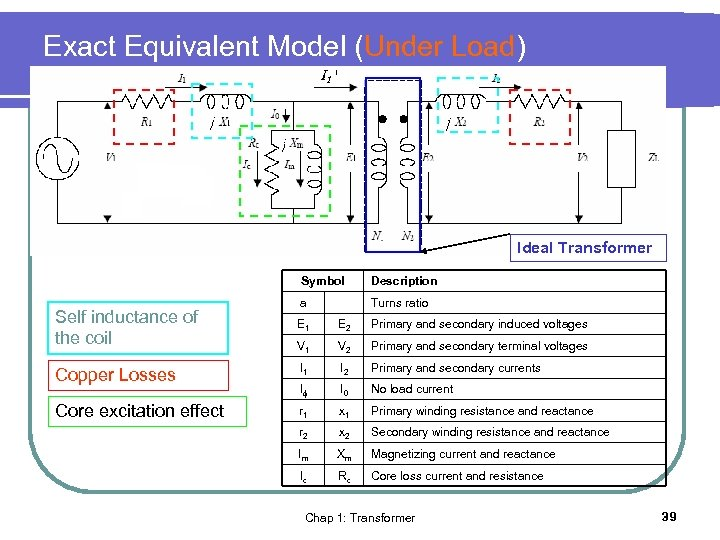Exact Equivalent Model (Under Load) Ideal Transformer Symbol Self inductance of the coil Description a Turns ratio E 1 E 2 Primary and secondary induced voltages V 1 V 2 Primary and secondary terminal voltages Copper Losses I 1 I 2 Primary and secondary currents I I 0 No load current Core excitation effect r 1 x 1 Primary winding resistance and reactance r 2 x 2 Secondary winding resistance and reactance Im Xm Magnetizing current and reactance Ic Rc Core loss current and resistance Chap 1: Transformer 39Exact Equivalent Model (No-Load) No-Load Power out = 0 (no load at secondary ) Power in = power out + power loss w Power loss = core loss + Cu loss h e Cu = 0 (no load) r e Power in = core loss b =Ic 2 Rc Watt y Chap 1: Transformer 40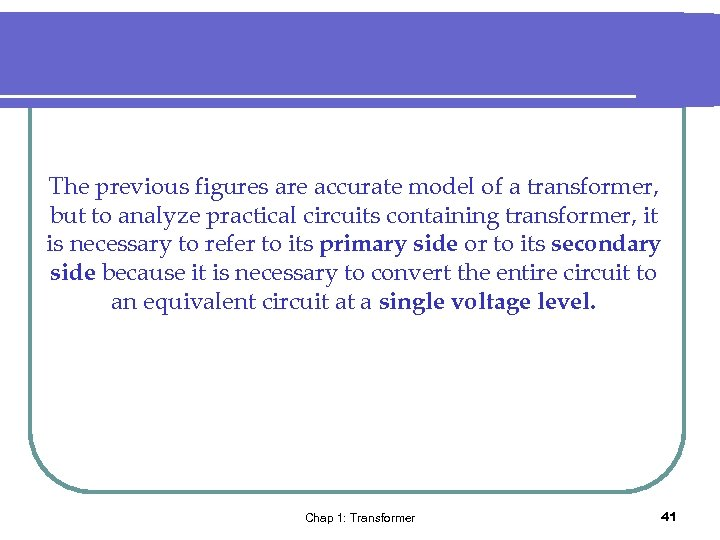The previous figures are accurate model of a transformer, but to analyze practical circuits containing transformer, it is necessary to refer to its primary side or to its secondary side because it is necessary to convert the entire circuit to an equivalent circuit at a single voltage level. Chap 1: Transformer 41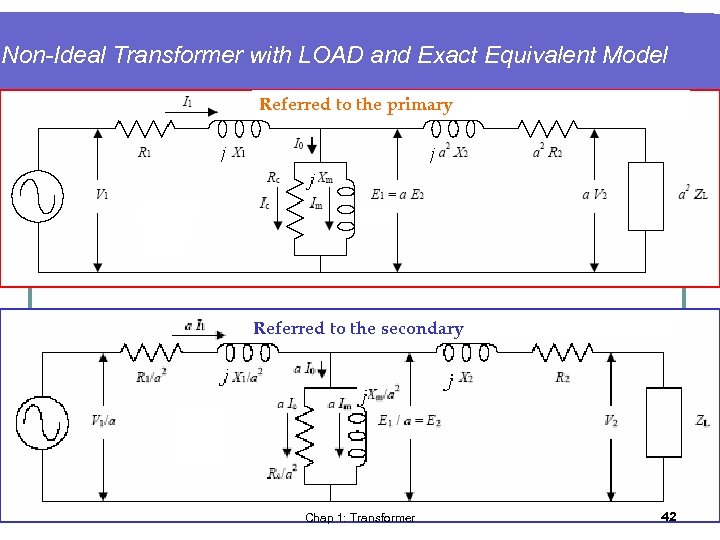Non-Ideal Transformer with LOAD and Exact Equivalent Model Referred to the primary Referred to the secondary Chap 1: Transformer 42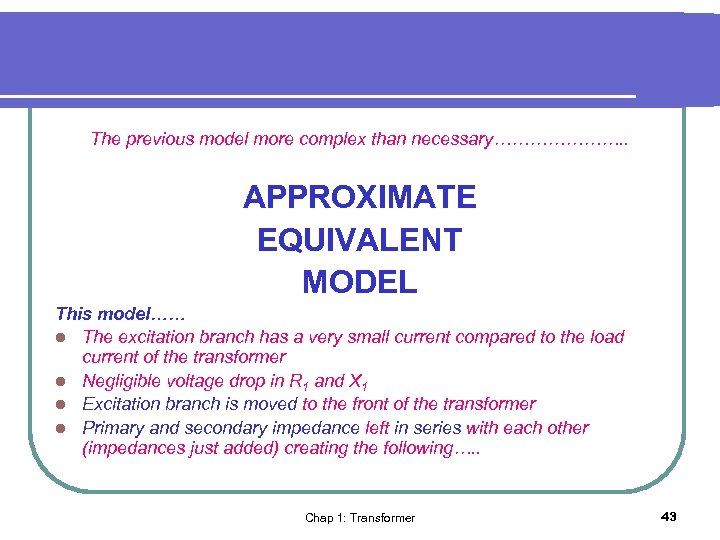The previous model more complex than necessary…………………. . APPROXIMATE EQUIVALENT MODEL This model…… l The excitation branch has a very small current compared to the load current of the transformer l Negligible voltage drop in R 1 and X 1 l Excitation branch is moved to the front of the transformer l Primary and secondary impedance left in series with each other (impedances just added) creating the following…. . Chap 1: Transformer 43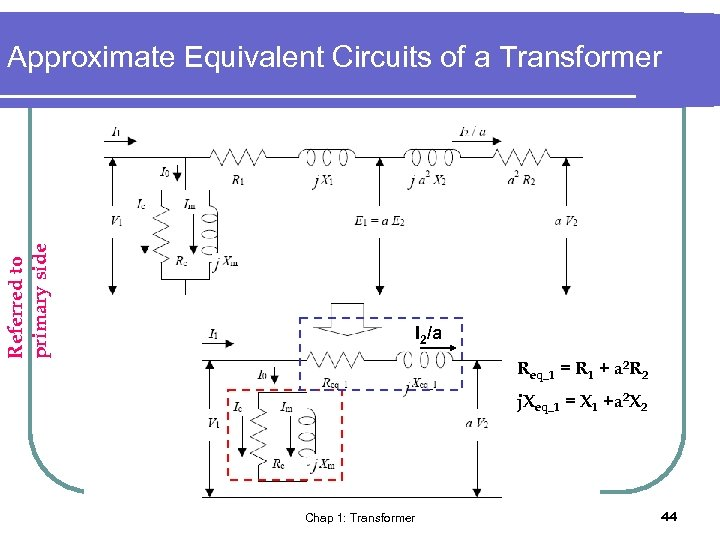Referred to primary side Approximate Equivalent Circuits of a Transformer I 2/a Req_1 = R 1 + a 2 R 2 j. Xeq_1 = X 1 +a 2 X 2 Chap 1: Transformer 44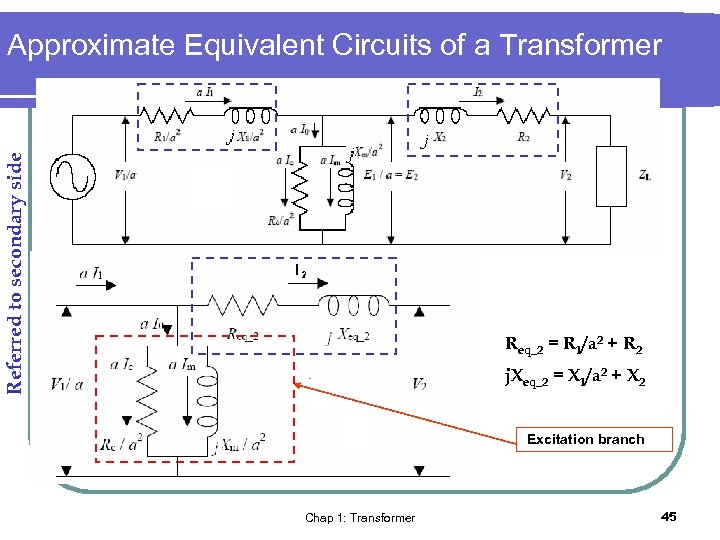Referred to secondary side Approximate Equivalent Circuits of a Transformer Req_2 = R 1/a 2 + R 2 j. Xeq_2 = X 1/a 2 + X 2 Excitation branch Chap 1: Transformer 45Exercise 1. 2 An ideal, single phase 2400 V-240 V transformer. The primary is connected to a 2200 V source and the secondary is connected to an impedance of 2Ω 36. 9°. a) Find the secondary output current and voltage. b) Find the primary input current. c) Find the load impedance as seen from the primary side. d) Find the input and output apparent powers. e) Find the output power factor. Chap 1: Transformer 46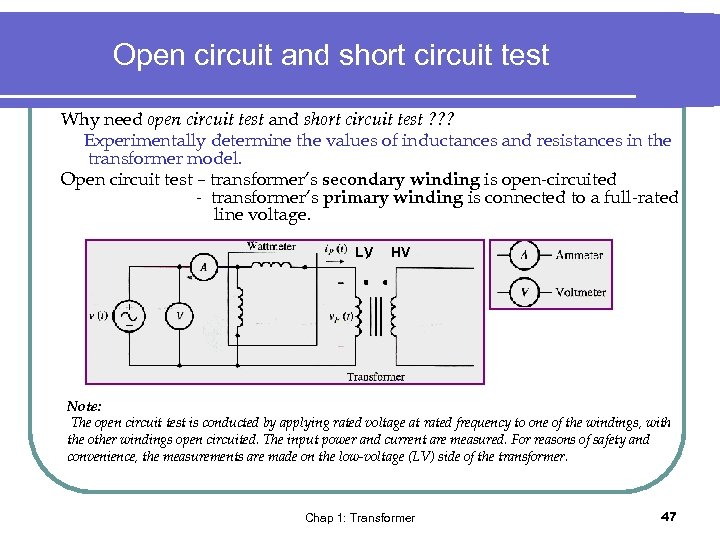Open circuit and short circuit test Why need open circuit test and short circuit test ? ? ? Experimentally determine the values of inductances and resistances in the transformer model. Open circuit test – transformer’s secondary winding is open-circuited - transformer’s primary winding is connected to a full-rated line voltage. LV HV Note: The open circuit test is conducted by applying rated voltage at rated frequency to one of the windings, with the other windings open circuited. The input power and current are measured. For reasons of safety and convenience, the measurements are made on the low-voltage (LV) side of the transformer. Chap 1: Transformer 47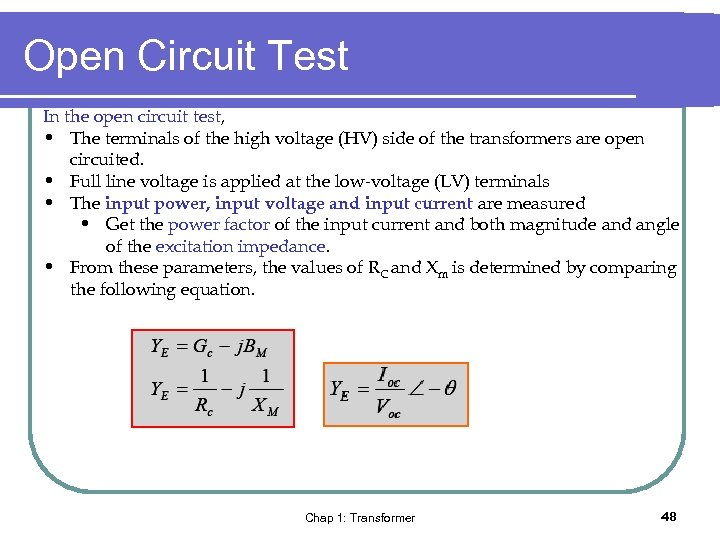Open Circuit Test In the open circuit test, • The terminals of the high voltage (HV) side of the transformers are open circuited. • Full line voltage is applied at the low-voltage (LV) terminals • The input power, input voltage and input current are measured • Get the power factor of the input current and both magnitude and angle of the excitation impedance. • From these parameters, the values of RC and Xm is determined by comparing the following equation. Chap 1: Transformer 48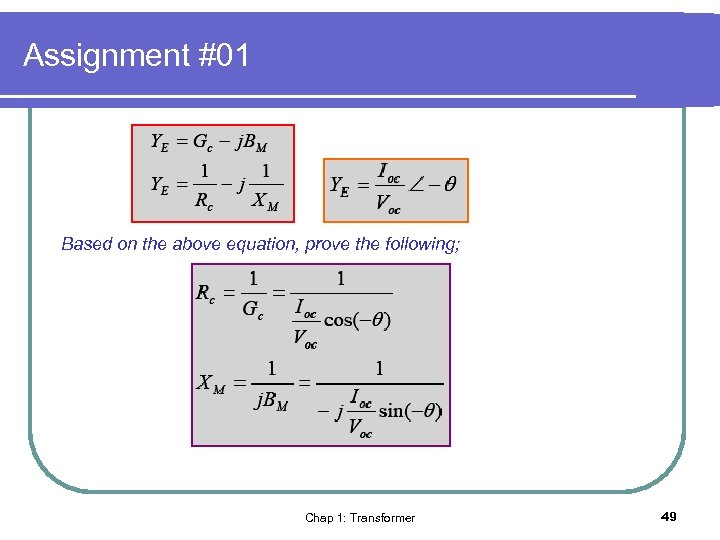Assignment #01 Based on the above equation, prove the following; Chap 1: Transformer 49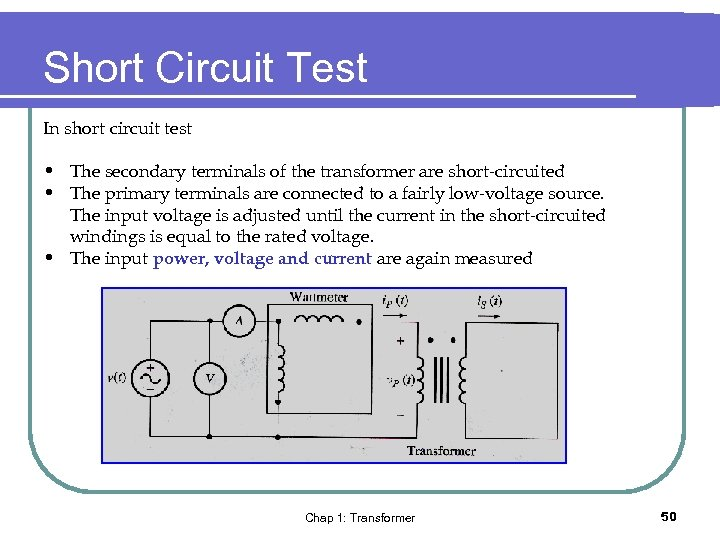Short Circuit Test In short circuit test • The secondary terminals of the transformer are short-circuited • The primary terminals are connected to a fairly low-voltage source. The input voltage is adjusted until the current in the short-circuited windings is equal to the rated voltage. • The input power, voltage and current are again measured Chap 1: Transformer 50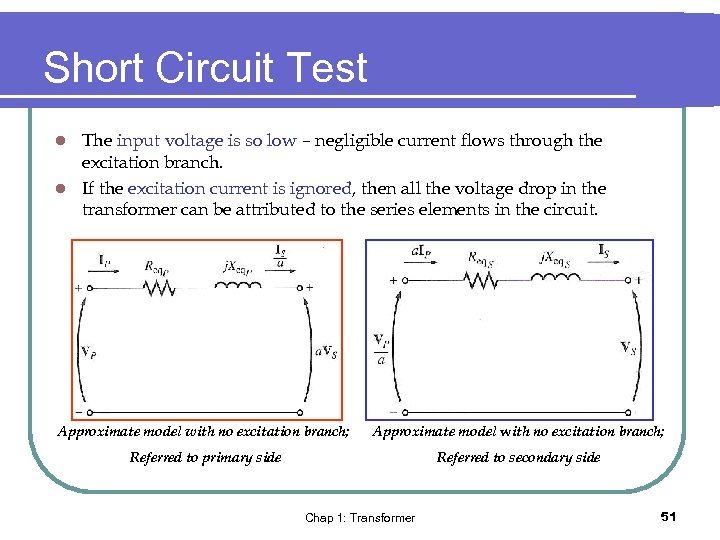Short Circuit Test The input voltage is so low – negligible current flows through the excitation branch. l If the excitation current is ignored, then all the voltage drop in the transformer can be attributed to the series elements in the circuit. l Approximate model with no excitation branch; Referred to primary side Referred to secondary side Chap 1: Transformer 51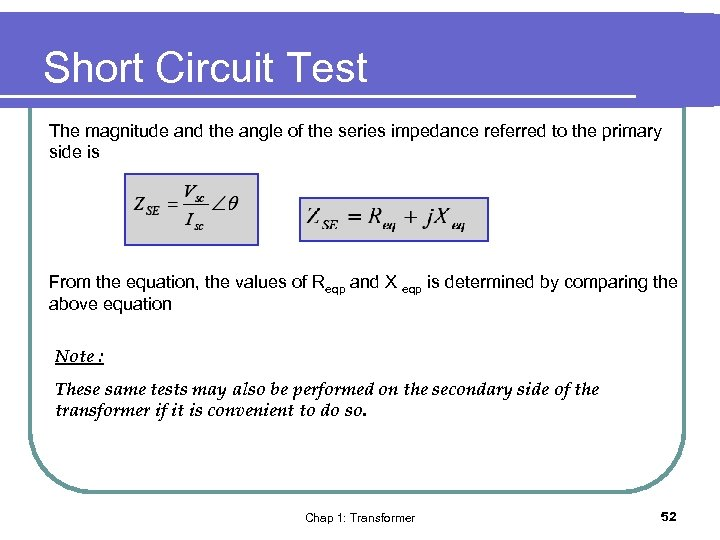Short Circuit Test The magnitude and the angle of the series impedance referred to the primary side is From the equation, the values of Reqp and X eqp is determined by comparing the above equation Note : These same tests may also be performed on the secondary side of the transformer if it is convenient to do so. Chap 1: Transformer 52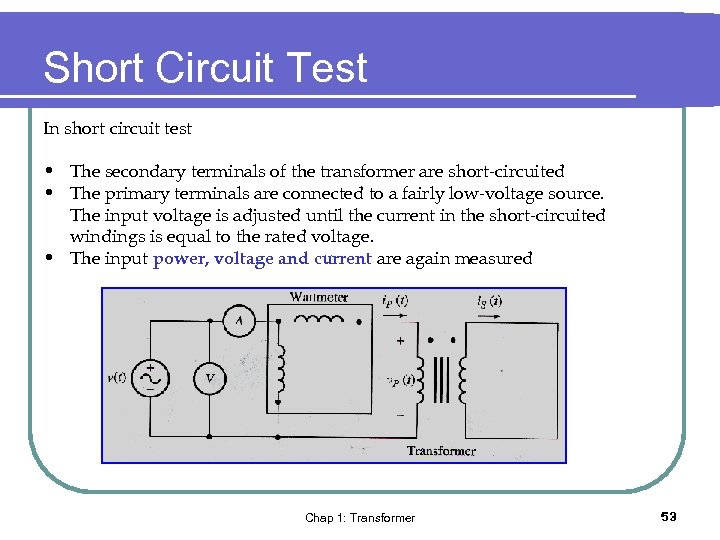Short Circuit Test In short circuit test • The secondary terminals of the transformer are short-circuited • The primary terminals are connected to a fairly low-voltage source. The input voltage is adjusted until the current in the short-circuited windings is equal to the rated voltage. • The input power, voltage and current are again measured Chap 1: Transformer 53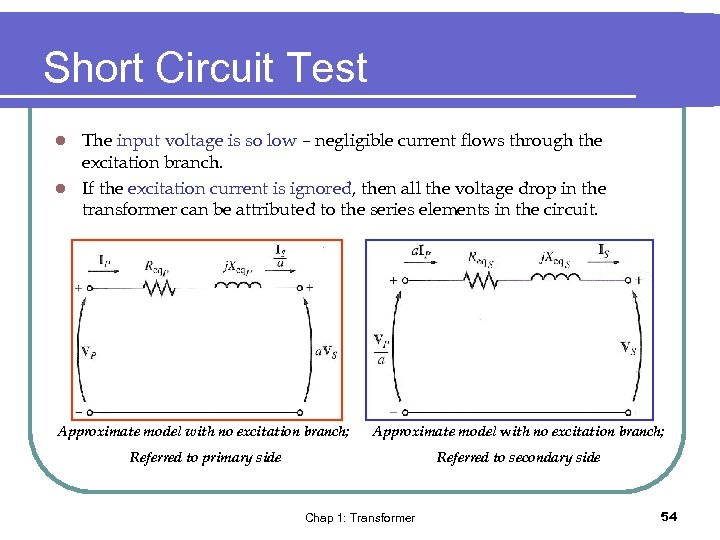Short Circuit Test The input voltage is so low – negligible current flows through the excitation branch. l If the excitation current is ignored, then all the voltage drop in the transformer can be attributed to the series elements in the circuit. l Approximate model with no excitation branch; Referred to primary side Referred to secondary side Chap 1: Transformer 54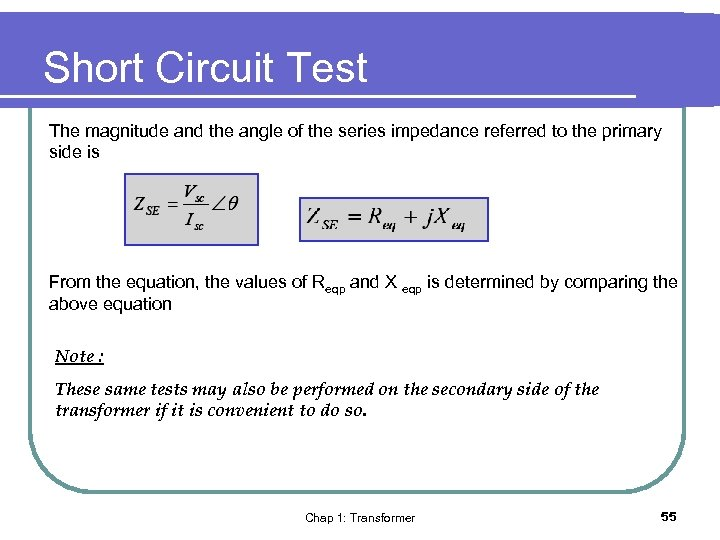Short Circuit Test The magnitude and the angle of the series impedance referred to the primary side is From the equation, the values of Reqp and X eqp is determined by comparing the above equation Note : These same tests may also be performed on the secondary side of the transformer if it is convenient to do so. Chap 1: Transformer 55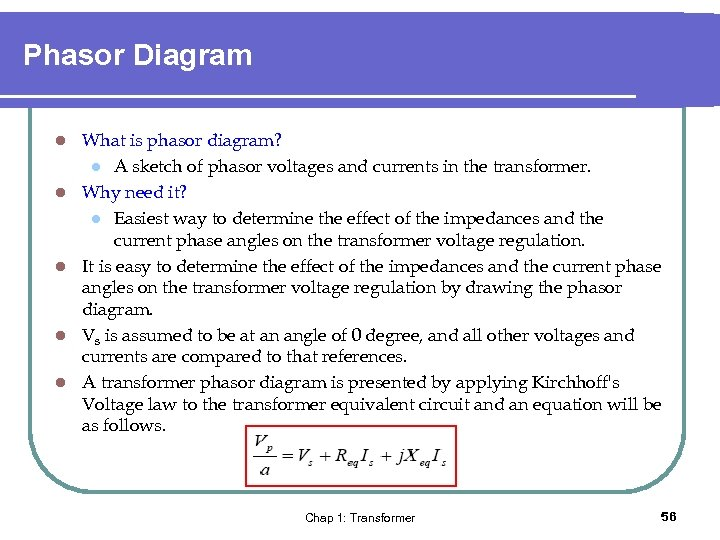Phasor Diagram l l l What is phasor diagram? l A sketch of phasor voltages and currents in the transformer. Why need it? l Easiest way to determine the effect of the impedances and the current phase angles on the transformer voltage regulation. It is easy to determine the effect of the impedances and the current phase angles on the transformer voltage regulation by drawing the phasor diagram. Vs is assumed to be at an angle of 0 degree, and all other voltages and currents are compared to that references. A transformer phasor diagram is presented by applying Kirchhoff's Voltage law to the transformer equivalent circuit and an equation will be as follows. Chap 1: Transformer 56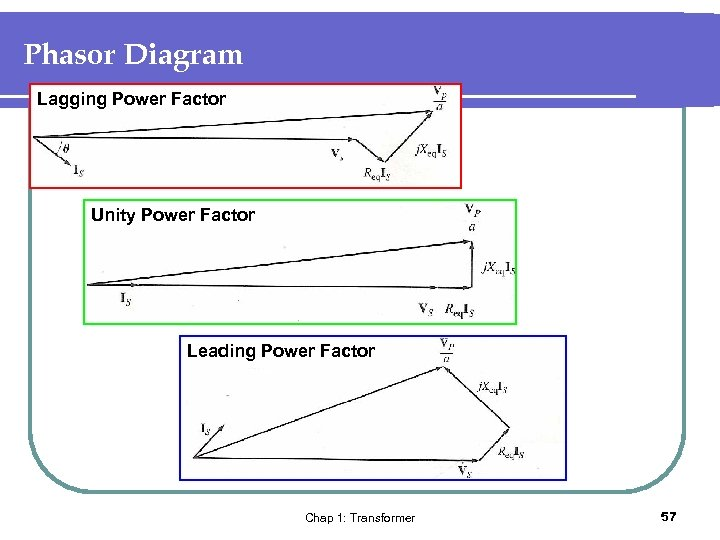Phasor Diagram Lagging Power Factor Unity Power Factor Leading Power Factor Chap 1: Transformer 57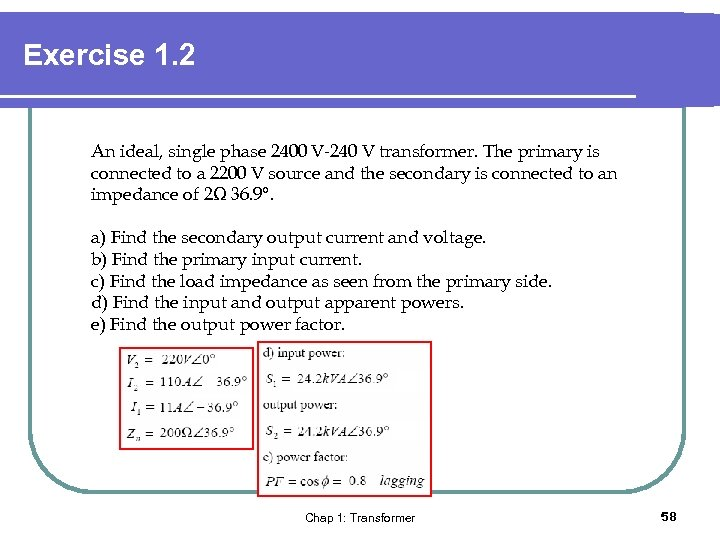Exercise 1. 2 An ideal, single phase 2400 V-240 V transformer. The primary is connected to a 2200 V source and the secondary is connected to an impedance of 2Ω 36. 9°. a) Find the secondary output current and voltage. b) Find the primary input current. c) Find the load impedance as seen from the primary side. d) Find the input and output apparent powers. e) Find the output power factor. Chap 1: Transformer 58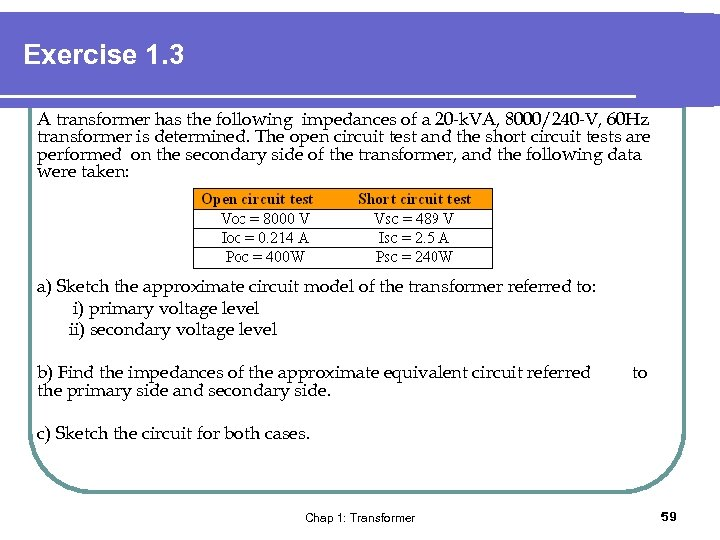Exercise 1. 3 A transformer has the following impedances of a 20 -k. VA, 8000/240 -V, 60 Hz transformer is determined. The open circuit test and the short circuit tests are performed on the secondary side of the transformer, and the following data were taken: a) Sketch the approximate circuit model of the transformer referred to: i) primary voltage level ii) secondary voltage level b) Find the impedances of the approximate equivalent circuit referred the primary side and secondary side. to c) Sketch the circuit for both cases. Chap 1: Transformer 59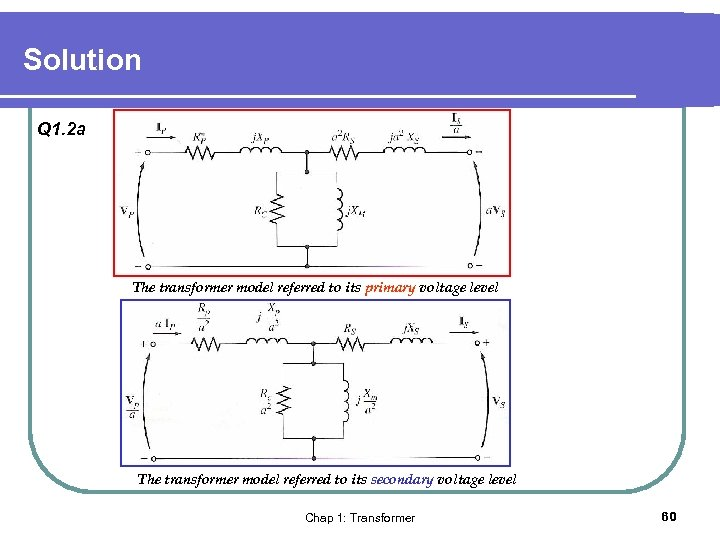Solution Q 1. 2 a The transformer model referred to its primary voltage level The transformer model referred to its secondary voltage level Chap 1: Transformer 60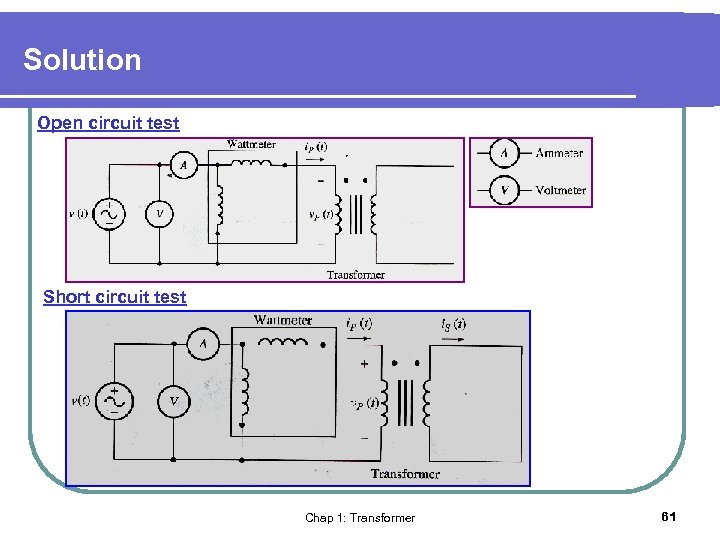Solution Open circuit test Short circuit test Chap 1: Transformer 61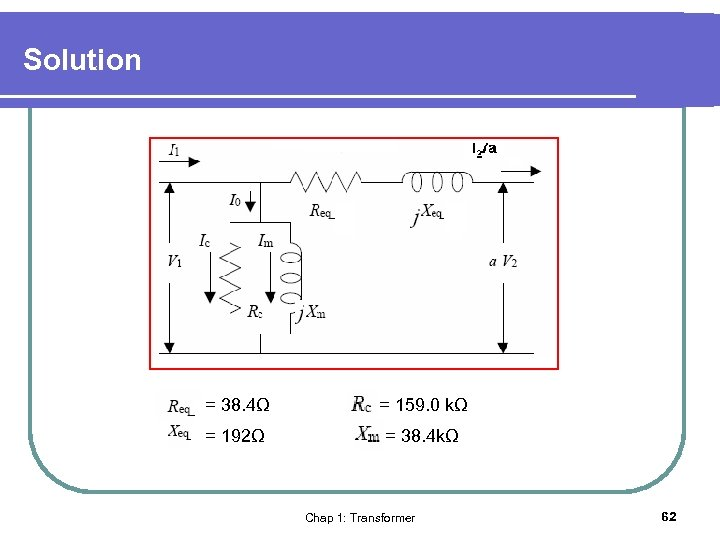Solution = 38. 4Ω = 159. 0 kΩ = 192Ω = 38. 4 kΩ Chap 1: Transformer 62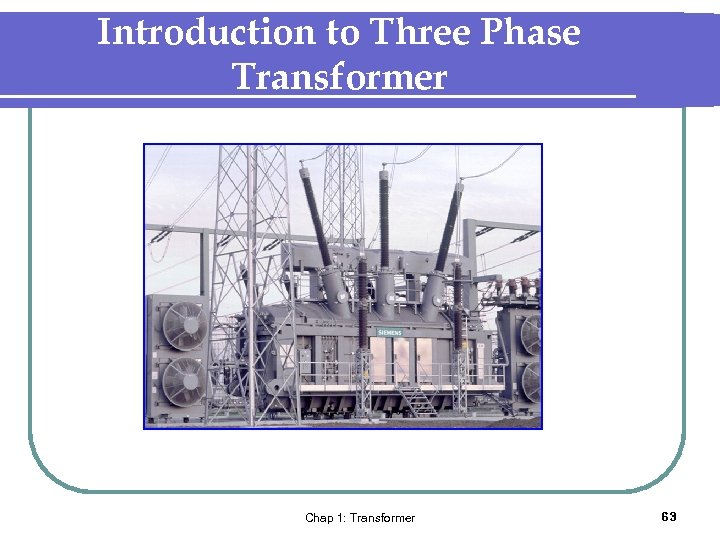Introduction to Three Phase Transformer Chap 1: Transformer 63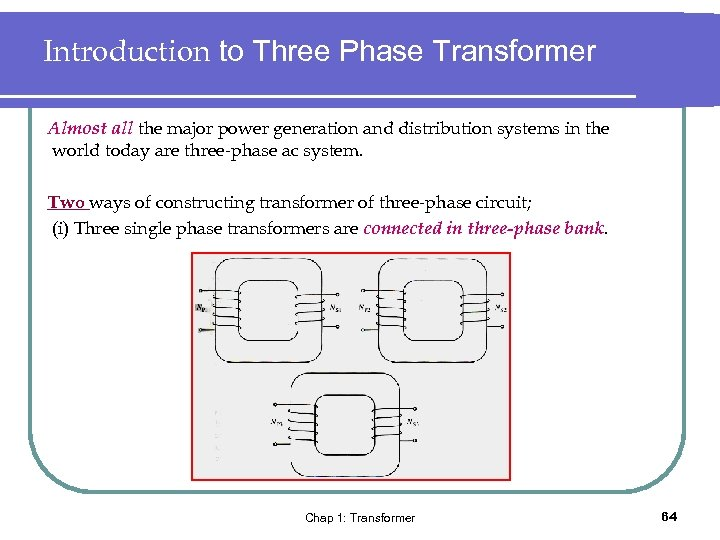Introduction to Three Phase Transformer Almost all the major power generation and distribution systems in the world today are three-phase ac system. Two ways of constructing transformer of three-phase circuit; (i) Three single phase transformers are connected in three-phase bank. Chap 1: Transformer 64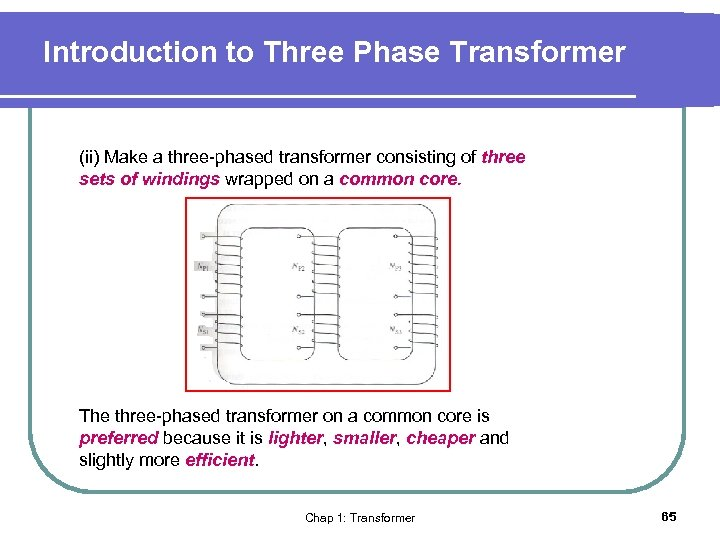Introduction to Three Phase Transformer (ii) Make a three-phased transformer consisting of three sets of windings wrapped on a common core. The three-phased transformer on a common core is preferred because it is lighter, smaller, cheaper and slightly more efficient. Chap 1: Transformer 65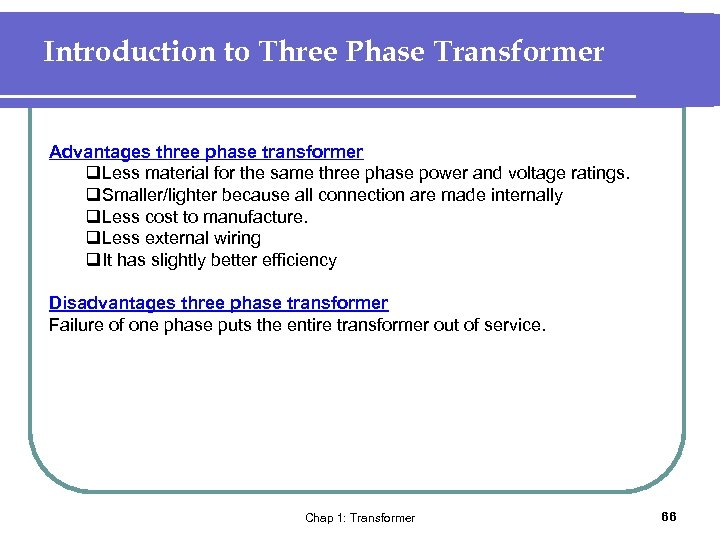Introduction to Three Phase Transformer Advantages three phase transformer q. Less material for the same three phase power and voltage ratings. q. Smaller/lighter because all connection are made internally q. Less cost to manufacture. q. Less external wiring q. It has slightly better efficiency Disadvantages three phase transformer Failure of one phase puts the entire transformer out of service. Chap 1: Transformer 66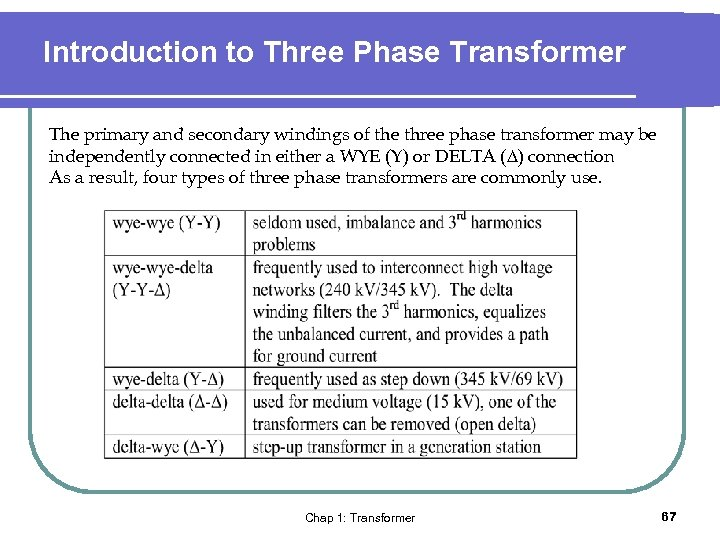Introduction to Three Phase Transformer The primary and secondary windings of the three phase transformer may be independently connected in either a WYE (Y) or DELTA ( ) connection As a result, four types of three phase transformers are commonly use. Chap 1: Transformer 67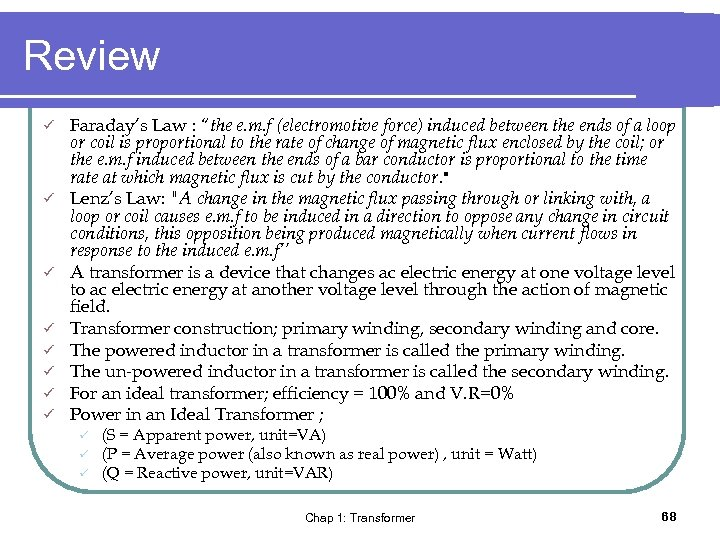Review ü ü ü ü Faraday’s Law : “the e. m. f (electromotive force) induced between the ends of a loop or coil is proportional to the rate of change of magnetic flux enclosed by the coil; or the e. m. f induced between the ends of a bar conductor is proportional to the time rate at which magnetic flux is cut by the conductor. " Lenz’s Law: "A change in the magnetic flux passing through or linking with, a loop or coil causes e. m. f to be induced in a direction to oppose any change in circuit conditions, this opposition being produced magnetically when current flows in response to the induced e. m. f’’ A transformer is a device that changes ac electric energy at one voltage level to ac electric energy at another voltage level through the action of magnetic field. Transformer construction; primary winding, secondary winding and core. The powered inductor in a transformer is called the primary winding. The un-powered inductor in a transformer is called the secondary winding. For an ideal transformer; efficiency = 100% and V. R=0% Power in an Ideal Transformer ; ü ü ü (S = Apparent power, unit=VA) (P = Average power (also known as real power) , unit = Watt) (Q = Reactive power, unit=VAR) Chap 1: Transformer 68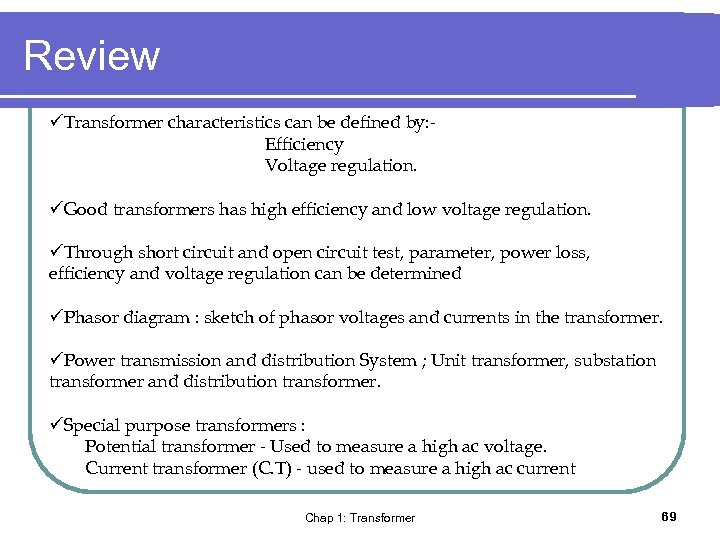Review üTransformer characteristics can be defined by: Efficiency Voltage regulation. üGood transformers has high efficiency and low voltage regulation. üThrough short circuit and open circuit test, parameter, power loss, efficiency and voltage regulation can be determined üPhasor diagram : sketch of phasor voltages and currents in the transformer. üPower transmission and distribution System ; Unit transformer, substation transformer and distribution transformer. üSpecial purpose transformers : Potential transformer - Used to measure a high ac voltage. Current transformer (C. T) - used to measure a high ac current Chap 1: Transformer 69